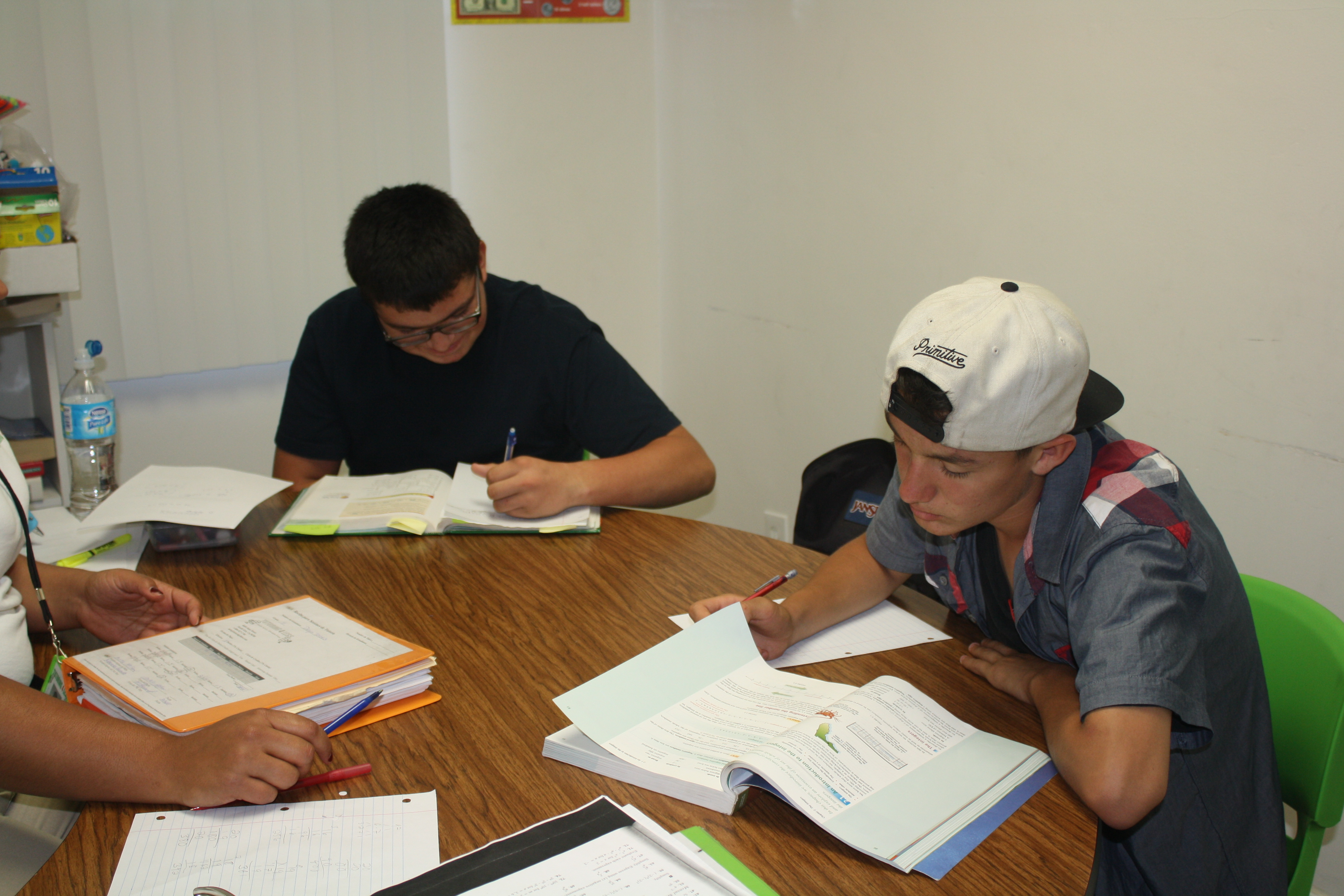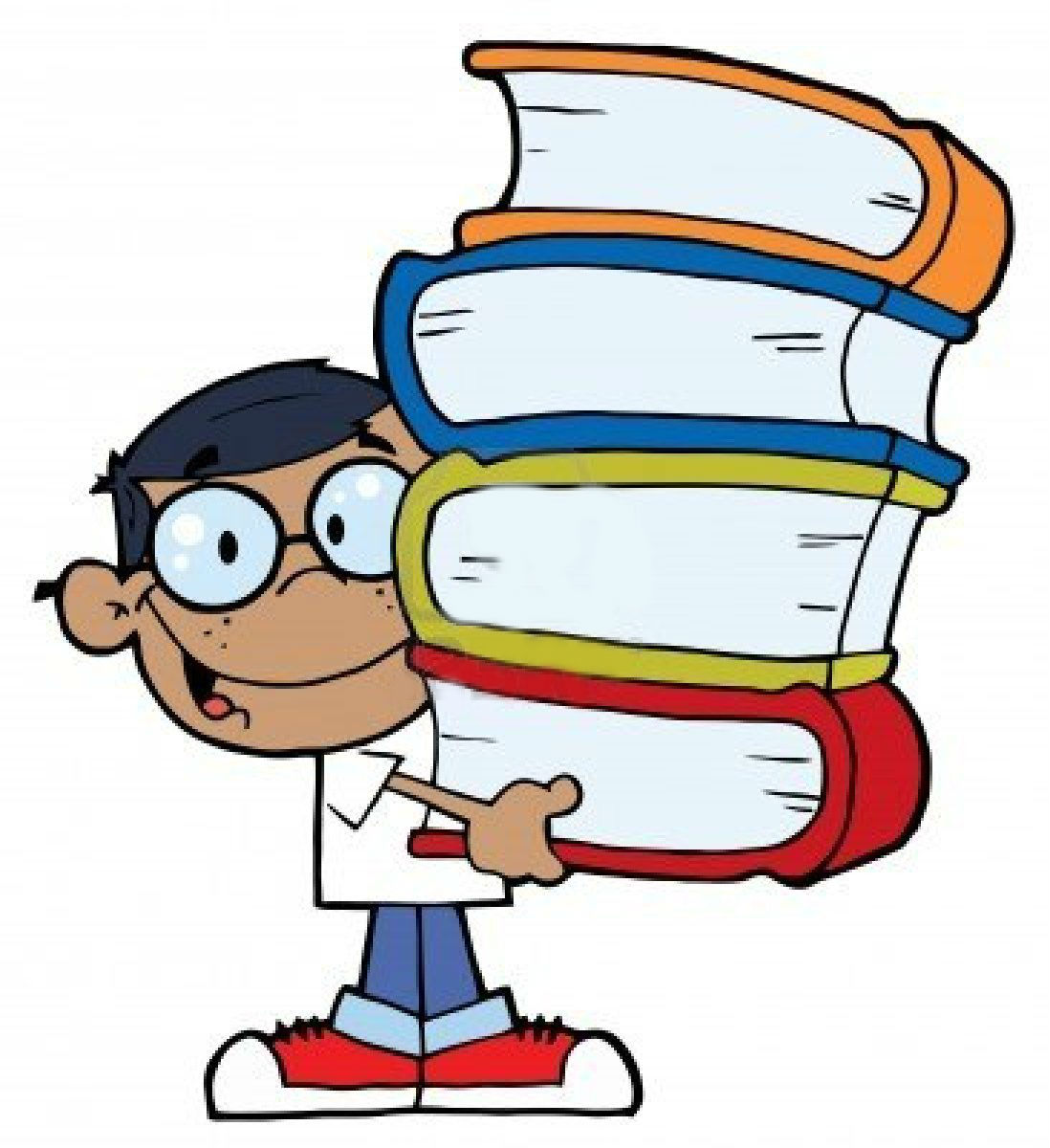#Seeking geometry tutoring for Los Angeles students? At Tutoring 4 Less we reinforce the concepts taught in High School Geometry by developing a detailed and foundation driven curriculum to meet each individual’s needs. We deepen the understanding of geometric relationships, spatial sense, angles, symmetry,  patterns, and help each student learn how to apply these concepts outside of the classroom. Tutors work with students in a small group or one on one to explore the properties and relationships between geometric figures such as triangles, quadrilaterals, and other polygons.

Having an advanced knowledge and understanding of the relationships between geometric objects and concepts help students apply these concepts to their everyday life. These learned concepts can be used to interpret by schematic drawings in architecture, estimating the amount of material needed for a construction project, rendering computer designs and graphics and even designing sewing patterns for the latest fashion trends.

## Online Tutoring For Geometry

Looking for math tutors whose teaching style is a good fit with your high school student can be trying at the best of times. Finding a private geometry tutor online can be even harder, when you’re not able to meet in person and establish a rapport, assess an individual’s credibility, and see if their teaching experience meshes with your student’s learning style. That’s why Tutoring 4 Less provides our private tutoring sessions, with our select group of qualified tutors. All our tutors have years of experience tutoring their chosen subject matter, be it reading, writing, chemistry, biology, pre-calculus, geometry, or even elementary school math and science. Our online tutoring program leverages the proven aspects of our in-person Los Angeles tutoring center, with the added benefits of online accessibility, any time, anywhere.

## Master Geometry With The Best Geometry Tutors in Los Angeles

Geometry is one of the five mathematical courses offered to students at the high school level.  Although most students are exposed to basic geometric relationships and schematics during their elementary education, it isn’t until high school that they begin to develop a more precise definition and understanding of the concepts taught in this class. At Tutoring 4 Less, our innovative geometry curriculum provides LA County students with both theoretical and experimental modeling tools that will allow them to apply geometric concepts to everyday life. A more detailed description of our curriculum can be studied below:

• Introduction to Geometry: This section reinforces the students understanding of points, lines, measuring, planes, and angles. They review how to graph on both a one-dimensional and two-dimensional plane. Students learn about the different geometric points and lines, such as: parallel and perpendicular lines, collinear and noncollinear points and planes, naming conventions, and angle relationships. Students review how to use a compass and protractor to measure and construct segments and shapes. They also review obtuse, acute, supplementary, complimentary, congruent, and right angles.
• Reasoning and Proving Theorems: This section of Geometry focuses on helping students organize their reasoning in a logical way. They learn about classifying different polygons and angles into a sequencing manner. Students are introduced to 3 types of reasoning techniques; conjecture, example and counter-example, and inductive and deductive reasoning. Students are introduced to the Geometric theorems that they will use throughout their mathematical careers to solve multi-step problems.
• Lines and Angles: Students learn about perpendicular and parallel lines, angles, slopes. They also learn how to solve and apply the midpoint and distance formulas.
• Triangles: Students learn about classifying triangles and solving their angles, sides, areas, and perimeters. They learn about isosceles, equilateral, right, acute, and obtuse triangles. They also learn how to use properties of exterior and interior angles to solve different problems and learn the triangle postulates and theorems. Students will also be exposed to the Pythagorean Theorem and how to use its application to solve for the different sides of a triangle.
• Circles, Polygons, and Quadrilaterals: Students are taught about perimeter, circumference, area, arc length, and geometric probability. They learn how to solve the area and perimeter for rectangles, squares, and parallelograms, and also find the circumference of circles.
• Transformations: In this section, students learn about translations and transformations of different geometric shapes within the x-y coordinate plane. They learn about degree rotation, asymptotes, reflections, dilation, and symmetry.
• Surface Area and Volume: Students are required to use formulas of surface area and volume for prisms, cylinders, cones, and pyramids. They also learn the different dimensions of geometric solids in order to compare the effects of altering the shapes dimensions.